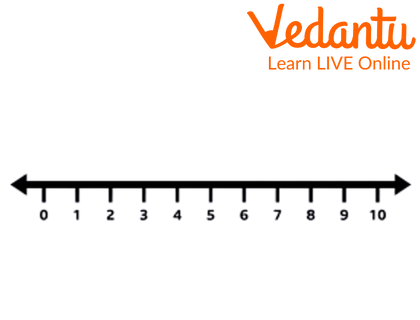Courses
Courses for Kids
Free study material
Free LIVE classes
More

# Class IntervalLIVE
Join Vedantu’s FREE Mastercalss

## What is Class Interval?

The Class interval is nothing but the difference between the upper-class limit and the lower-class limit. For example, if the lower limit is 5 and the upper limit is 8 then, the class interval will be 4, i.e, the difference between the upper limit and the lower limit. In this article, we will discuss class intervals in detail.Class Interval

## Intervals in Maths

The intervals in Maths are measured in the number. it includes the number which comes between two particular defined numbers. It cannot be defined in terms of the kind or any non-measurable factor. it should be in number only.Number Line

The numbers that mark from left to right of the intervals are called endpoints of the intervals; it may or may not be included in the intervals. The endpoints intervals can be included and cannot be included. It all depends on how you are going to collect or the requirements made by the organisation. Here, it all depends upon the given question, if it’s mentioned that it is included, then it should be included in the class interval otherwise not.

## Types of Intervals in Maths

The types of intervals are the following:

• Open Interval

• Closed Interval

• Half Bounded Interval

• Bounded Set

## How to Calculate Class Interval?

The class interval can be calculated by the difference between the upper-class limit and the lower-class limit.

## Example Question:

Example 1: Given is the frequency distribution that represents the number of wins by a cricket team:

 Wins Frequency 26 – 30 2 31 – 35 3 36 – 40 7 41 – 45 8 46 – 50 4 51 – 55 3 56 - 60 2

Ans: Class interval = Upper-class limit – Lower-class limit

 Wins Frequency Lower Class Limit Upper Class Limit 26 - 30 2 26 30 31 – 35 3 31 35 36 – 40 7 36 40 41 – 45 8 41 45 46 – 50 4 46 50 51 – 55 3 51 55 56 - 60 2 56 60

So, in this given question, the lowest numbers in wins is the lower limit and the highest number is the upper class limit.

Class interval $= {\rm{ }}30{\rm{ }}-{\rm{ }}26{\rm{ }} = 4$

Or $35{\rm{ }}-{\rm{ }}31{\rm{ }} = {\rm{ }}4{\rm{ }}.$

All the class intervals will be 4.

Example 2: Calculate the class interval of the following table:

 Units Frequency 21 – 30 5 31 - 40 8

Ans: Class interval = Upper-class limit – Lower-class limit

$= {\rm{ }}30{\rm{ }}-{\rm{ }}21$

$= {\rm{ }}9$

## Solved Questions

1. Calculate the class interval if the upper limit is 85 and the lower limit is 45.

Ans: Class interval = Upper-class limit – Lower-class limit

$= {\rm{ }}85{\rm{ }}-{\rm{ }}45$

$= {\rm{ }}40$

2. What is the lower limit if the class interval is 50 and the upper limit is 98?

Ans: Class interval = Upper-class limit – Lower-class limit

$50{\rm{ }} = {\rm{ }}98{\rm{ }}-$lower limit

Lower limit $= {\rm{ }}98{\rm{ }}-{\rm{ }}50$

Lower limit $= {\rm{ }}48.$

3. Calculate the class interval if the upper limit is 87.25 and the lower limit is 65.45.

Ans: Class interval = Upper-class limit – Lower-class limit

$= {\rm{ }}87.25{\rm{ }}-{\rm{ }}65.45$

$= {\rm{ }}21.8$

## Summary

In this topic, we have learned about the class interval. Class interval is the difference between the upper limit and the lower limit. Class interval in Maths is always represented in the number. The formula for the class interval is Class interval = Upper-class limit – Lower class limit.

Last updated date: 23rd Sep 2023
Total views: 100.5k
Views today: 2.00k

## FAQs on Class Interval

1. Give an example of  a class?

The height of the students in a class. For Example, 50-60 kg.

2. How are the statistics used in real life?

The statistics are used in the real-life world. For example, to calculate the census, to know the sales report etc.

3. Give an example of an open interval.

The open end interval does include the endpoint. Example: 40-50 in this 40 and 50 are not included in the interval.